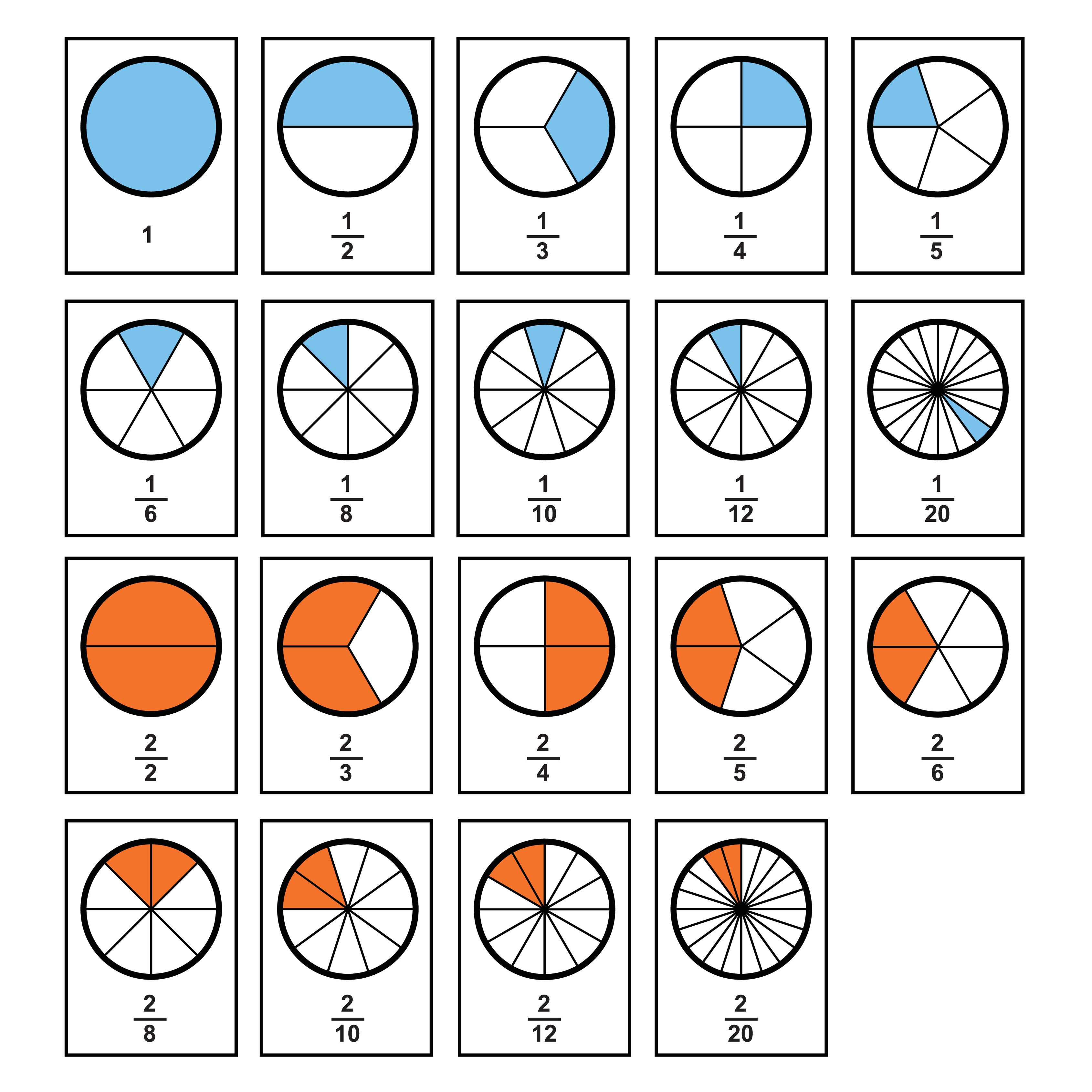What is a fraction?

A fraction represents a part of a whole. It is made up of a numerator on the top and a denominator on the bottom.

The bottom of a fraction (the denominator) is the number of equal parts in a whole.

For example, if we have a cake and we divide it into 4 equal slices the denominator would be 4 because the whole cake is made up of 4 slices.

The top of a fraction (the numerator) is the number of those equal parts we are talking about.

For example, if we give two of our friends a slice of cake the numerator would be 2 because we have given 2 slices out of 4 away.

Here are some examples of fractions of a group:We use a line to divide the top and bottom of a fraction: 2/4